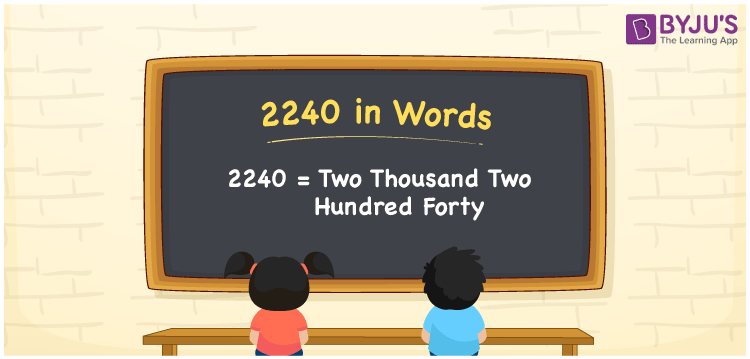# 2240 in Words

2240 in words can be written as Two Thousand Two Hundred Forty. Students will be able to learn the conversion of 2240 in words which will help them understand the applications of numbers in our daily lives. If you buy an iron box for Rs. 2240, then you can say that “I have bought an iron box for Two Thousand Two Hundred Forty Rupees”. The  number 2240 can be written in words using the English alphabet. The numbers in words can be grasped easily by the students using the resources given at BYJU’S. 2240 in English can be read as “Two Thousand Two Hundred Forty”.

 2240 in words Two Thousand Two Hundred Forty Two Thousand Two Hundred Forty in Numbers 2240

## 2240 in English Words## How to Write 2240 in Words?

Students will learn about the conversion of 2240 into words from place value charts. The number 2240 has four digits. For 2240, the place value chart is prepared in a table form to help students understand it effectively.

 Thousands Hundreds Tens Ones 2 2 4 0

2240 in expanded form is explained in brief here:

2 × Thousand + 2 × Hundred + 4 × Ten + 0 × One

= 2 × 1000  + 2 × 100 + 4 × 10 + 0 × 1

= 2000 + 200 + 40 + 0

= 2240

= Two Thousand Two Hundred Forty

Therefore, 2240 in words is written as Two Thousand Two Hundred Forty.

2240 is a natural number that precedes 2241 and succeeds 2239.

2240 in words – Two Thousand Two Hundred Forty

Is 2240 an odd number? – No

Is 2240 an even number? – Yes

Is 2240 a perfect square number? – No

Is 2240 a perfect cube number? – No

Is 2240 a prime number? – No

Is 2240 a composite number? – Yes

## Frequently Asked Questions on 2240 in Words

Q1

### How do you write 2240 in words?

2240 can be written as “Two Thousand Two Hundred Forty” in words.
Q2

### Is 2240 an even number?

2240 is an even number because it is divisible by 2.
Q3

### How can Two Thousand Two Hundred Forty be written in numbers?

Two Thousand Two Hundred Forty can be written in numbers as 2240.